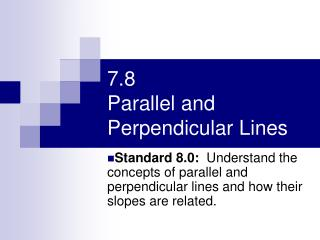DownloadDownload Presentation7.8 Parallel and Perpendicular Lines

# 7.8 Parallel and Perpendicular Lines

Télécharger la présentation## 7.8 Parallel and Perpendicular Lines

- - - - - - - - - - - - - - - - - - - - - - - - - - - E N D - - - - - - - - - - - - - - - - - - - - - - - - - - -
##### Presentation Transcript

1. 7.8 Parallel and Perpendicular Lines Standard 8.0: Understand the concepts of parallel and perpendicular lines and how their slopes are related.

2. Vocabulary • Parallel Lines– Lines that never cross and never intersect. • Perpendicular Lines – Lines that, when they cross each other, form right angles.

3. Steps for determining if graphs are parallel or perpendicular • Put both equations into slope-intercept form. • Find the slope and y-intercept of each equation. • Analyze the slope and y-intercept Parallel – Slope is the same; y-intercept different Perpendicular– Slope is a negative reciprocal

4. -3 1/3 -2/5 6 -3/2 -1/6 8 3/8 7/3 1/3 -3 5/2 -1/6 2/3 6 -1/8 -8/3 -3/7 Finding Opposite Reciprocals

5. #1 Determine whether the graphs of the equations are parallel lines

6. #2 Determine whether the graphs of the equations are parallel lines

7. #3 Determine whether the graphs of the equations are perpendicular lines

8. #4 Determine whether the graphs of the equations are perpendicular lines

9. #5 Write an equation for the line containing the given point and perpendicular to the given line.